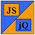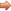# JavaScript & jQuery Tutorials

JS HomeJS AdvancedFunction Recursion & Closures

## Function Recursion & Closures

This lesson is about function recursion and function closures, what each means and how we can take advantage of these techniques.

### Function Recursion

Function recursion occurs when a function is referred to and called internally by itself. We can make a function refer to itself by using:

1. The function name
2. An in-scope variable which refers to the function

Let's take a look at each of these with some example code.

#### Function Recursion Using Function Name

The following function takes one input parameter and does a countdown from that parameter.

``````
// Simple countdown using recursion.
function countdown(a) {
if (a == 0)
return;
countdown(a - 1); // recursive call
}
countdown(3);

``````

Press the button below to action the above code:

#### Function Recursion Using An in-scope Variable

The following function defined within a variable takes one input parameter and does a countdown from that parameter.

``````
// Simple countdown using recursion with inscope avariable.
var aVariable = function countdown(a) {
if (a == 0)
return;
countdown(a - 1); // recursive call
}
aVariable(3);

``````

Press the button below to action the above code:

### Closures

So what is a closure?

A closure is created when an inner function accesses the arguments and variables of its outer function and is made available outside the function that called it.

The Closure definition needs some verification and beefing out, along with some rules. So lets show examples and expand on the definition as we go.

#### Closure Scope

Firstly lets get comfortable with inner and outer closure scope by looking at some rules and an example:

• The outer function has no access to any of the arguments and variables of the inner function.
• The inner function has access to all the arguments and variables of the outer function.

``````
// Looking at function scope.
function outer(x) {
funcScopeArray = 'outer function scope - x =: ' + x + '\n';
function inner(y) {
funcScopeArray = 'inner function scope - x =: ' + x
+ ', y =: ' + y + '\n';
function core(z) {
funcScopeArray = 'core function Scope - x =: ' + x
+ ', y =: ' + y + ', z =: ' + z;
}
core(3);
}
inner(2);
}
var funcScopeArray = new Array(3);
outer(1);

``````

Press the button below to action the above code:

As you can see when you press the button, the `Inner()` function has access to the scope of the `Outer()` function.

The `Core()` function has access to the scope of the `Inner()` function which encompasses the scope of the `Outer()` function as well.

If you run the code through the Firefox debugger you will see closure scopes being created as you step into the nested functions. They disappear when the function ends as we are not returning anything for later use.

• Technically a closure scope is created as soon as an inner function accesses an outer functions variables regardless of whether the inner function is used outside the function that called it. BUT the closure disappears as soon as the inner function ends, unless made available.

#### Forming A Persistent Closure

Now we have looked at closure scope lets go back to our definition above and form a persistent closure that we can utilize after our functions have ended.

``````
// Forming A Persistent Closure.
function outer(num) {
var x = num;
var scope = function inner(y) { return x + y; };
/*
* Here we return the variable which holds the function inner().
* This function references a variable from the outer() function (x).
* Which means we have created a persistent closure where we have access
* to x.
*/
return scope;
}
var aClosure = outer(10);
var bClosure = outer(20);
alert(aClosure + '\n' + bClosure); // Our returned closures
var a = aClosure();
var b = aClosure(5);
var c = aClosure(10);
var d = bClosure();
var e = bClosure(5);
var f = bClosure(10);
alert('a=' + a + ' b=' + b + ' c=' + c + ' d=' + d + ' e=' + e + ' f=' + f);

``````

Press the button below to action the above code:

We have returned the `Inner()` function as the variables `aClosure` and `bClosure` thus creating 2 persistent closures. When we created our closures we passed different values to the `Outer()` function each time we created them. After returning we called the variables `aClosure` and `bClosure` seperately and they returned values encompassing the original values passed to the `Outer()` function. This shows that every time we call the `Inner()` function closure a new set of local variables are created. So we can expand on our defintion with some new rules:

• A closure retains the set of local variables used in the creation of the closure.
• Each time a closure is called a new set of local variables are created.

#### Looking At Closure Local Variables

So what happens when we increment a variable passed to our `Inner()` function from the `Outer()` function after the `Inner()` function has run?

``````
function outer(num) {
var x = num;
var scope = function inner(y) { return x + y; };
x++; // Increment outer() function variable
return scope;
}
var aClosure = outer(10);
var a = aClosure();
var b = aClosure(5);
var c = aClosure(10);
alert('a=' + a + ' b=' + b + ' c=' + c);

``````

Press the button below to action the above code:

When we access our returned closure and apply some values we can see the results include the increment to the variable `x`. This shows that the set of local variables are held by reference and not just copied. So we can expand on our defintion with a new rule:

• A closures local variables are stored by reference and not copied.

#### Our Final Closure Definition

A closure is created when an inner function accesses the arguments and variables of its outer function and is made available outside the function that called it.

Technically a closure scope is created as soon as an inner function accesses an outer functions variables regardless of whether the inner function is used outside the function that called it. BUT the closure disappears as soon as the inner function ends, unless made available.

• A closure retains the set of local variables used in the creation of the closure.
• Each time a closure is called a new set of local variables are created.
• A closures local variables are stored by reference and not copied.

## Lesson 8 Complete

In this lesson we looked at function recursion and closures.

## Related Tutorials

JavaScript Intermediate Tutorials - Lesson 8 - Functions

## What's Next?

In the next lesson we wrap up our lessons on JavaScript with a look at the globals and statements not covered in previous lessons.